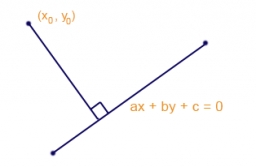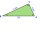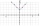# Find the 5

Find the equation with center at (1,20) which touches the line 8x+5y-19=0

Result

e = (Correct answer is: e = pow(x-1, 2)+pow(y-20, 2) = 89)#### Solution:We would be very happy if you find an error in the example, spelling mistakes, or inaccuracies, and please send it to us. We thank you!

Showing 1 comment:Dr Math
Hint - use formula for Distance Between a Point and a Line = which is radius of circle

2 years ago  2 LikesTips to related online calculators
For Basic calculations in analytic geometry is helpful line slope calculator. From coordinates of two points in the plane it calculate slope, normal and parametric line equation(s), slope, directional angle, direction vector, the length of segment, intersections the coordinate axes etc.
Two vectors given by its magnitudes and by included angle can be added by our vector sum calculator.
Pythagorean theorem is the base for the right triangle calculator.

#### You need to know the following knowledge to solve this word math problem:

We encourage you to watch this tutorial video on this math problem:

## Next similar math problems:

• Vector v4Find the vector v4 perpendicular to vectors v1 = (1, 1, 1, -1), v2 = (1, 1, -1, 1) and v3 = (0, 0, 1, 1)
• Rectangle 39Find the perimeter and area of the rectangular with vertices (-1, 4), (0,4), (0, -1), and (-4, 4)
• Coordinates of square verticesThe ABCD square has the center S [−3, −2] and the vertex A [1, −3]. Find the coordinates of the other vertices of the square.
• Three pointsThree points K (-3; 2), L (-1; 4), M (3, -4) are given. Find out: (a) whether the triangle KLM is right b) calculate the length of the line to the k side c) write the coordinates of the vector LM d) write the directional form of the KM side e) write the d
• The modulusFind the modulus of the complex number 2 + 5i
• TriangleTriangle KLM is given by plane coordinates of vertices: K[11, -10] L[10, 12] M[1, 3]. Calculate its area and its interior angles.
• On a lineOn a line p : 3 x - 4 y - 3 = 0, determine the point C equidistant from points A[4, 4] and B[7, 1].
• 3d vector componentThe vector u = (3.9, u3) and the length of the vector u is 12. What is is u3?
• Calculate 6Calculate the distance of a point A[0, 2] from a line passing through points B[9, 5] and C[1, -1].
• DodecagonCalculate the size of the smaller of the angles determined by lines A1 A4 and A2 A10 in the regular dodecagon A1A2A3. .. A12. Express the result in degrees.
• Angle of the body diagonalsUsing vector dot product calculate the angle of the body diagonals of the cube.An airplane leaves an airport and flies to west 120 miles and then 150 miles in the direction S 44.1°W. How far is the plane from the airport (round to the nearest mile)?Vector a has coordinates (8; 10) and vector b has coordinates (0; 17). If the vector c = b - a, what is the magnitude of the vector c?Determine coordinates of unit vector to vector AB if A[-6; 8], B[-18; 10].Calculate length of the vector v⃗ = (9.75, 6.75, -6.5, -3.75, 2).Points A[-9,7] and B[-4,-5] are adjacent vertices of the square ABCD. Calculate the area of the square ABCD.Passenger car and an ambulance come to the rectangular crossroad, the ambulance left. Passenger car at speed 39 km/h and ambulance at 41 km/h. Calculate such a relative speed of the ambulance move to the car.